Miscellaneous

Chapter 13 Class 12 Probability
Serial order wise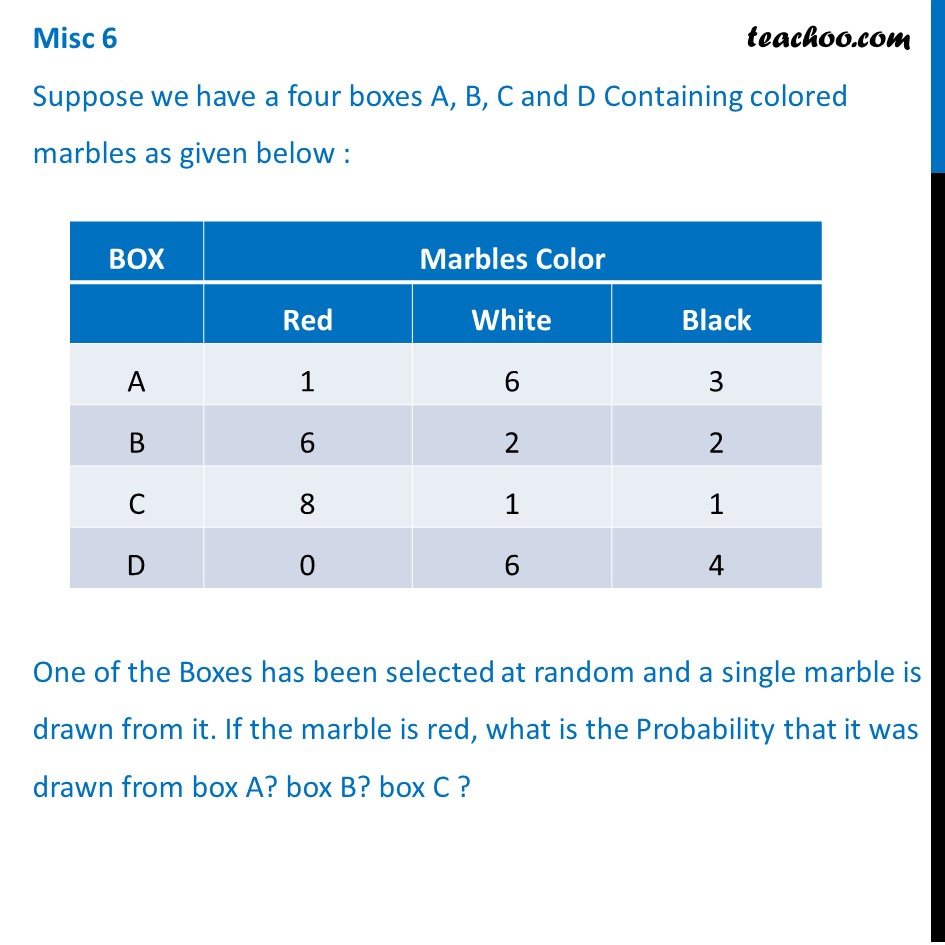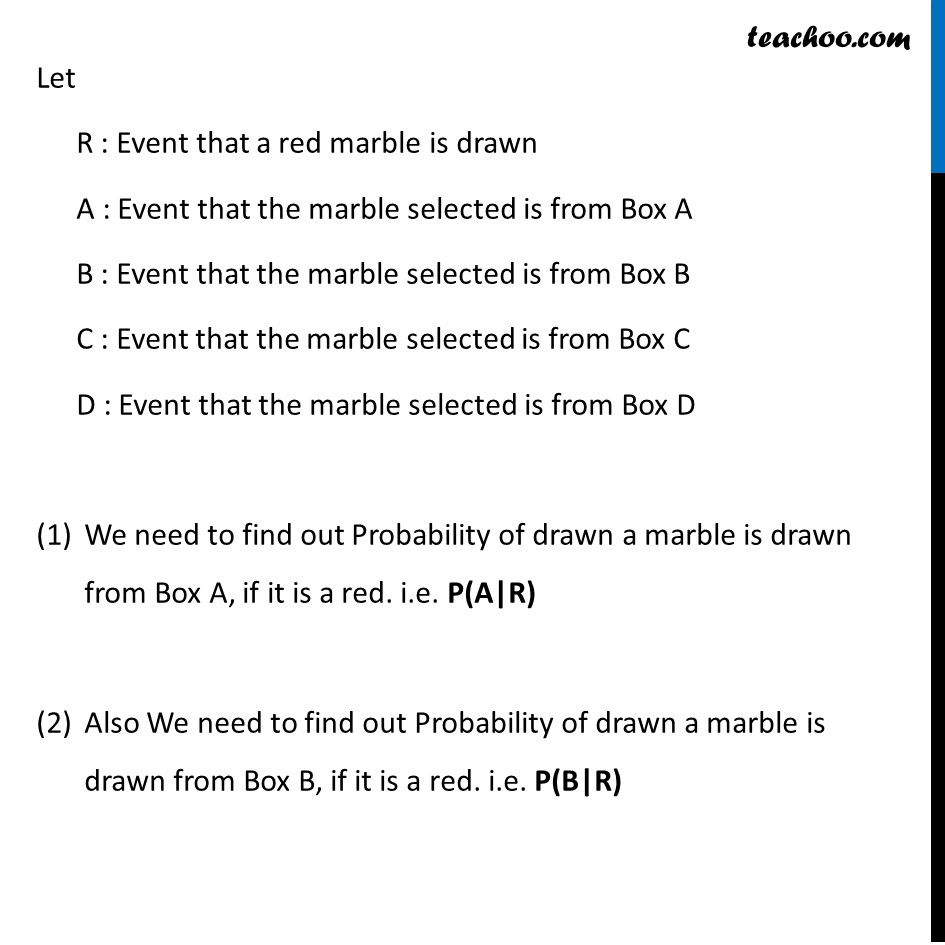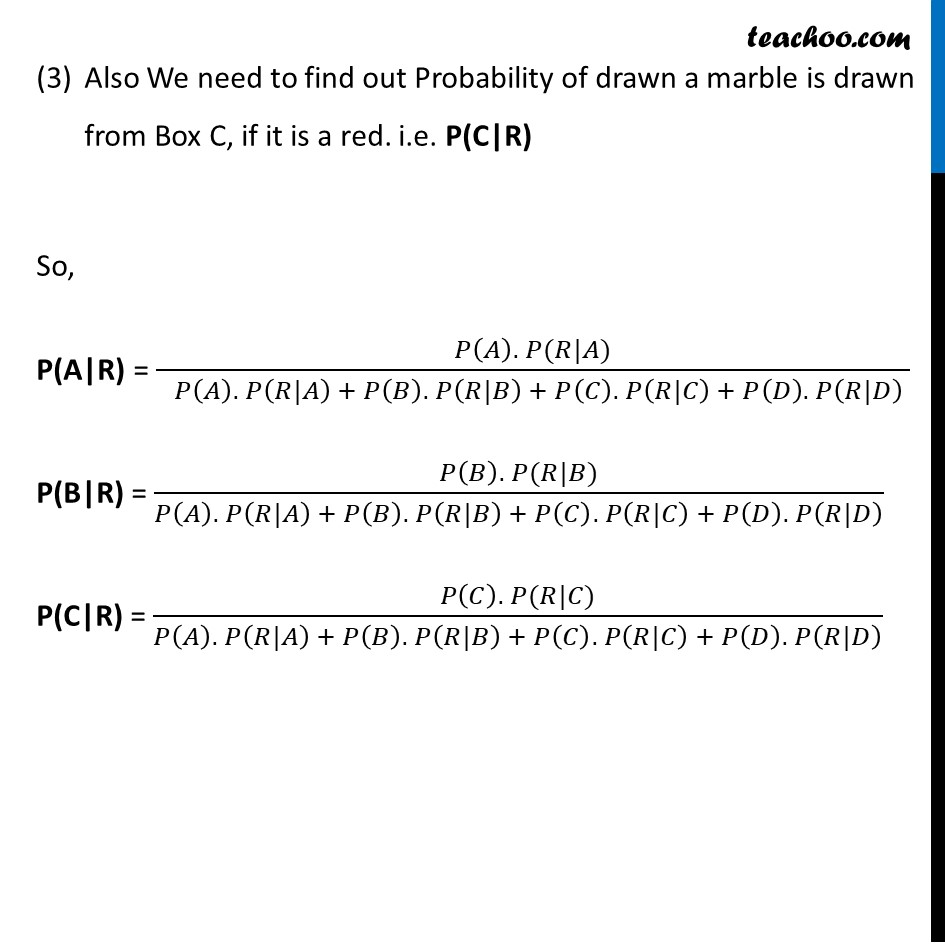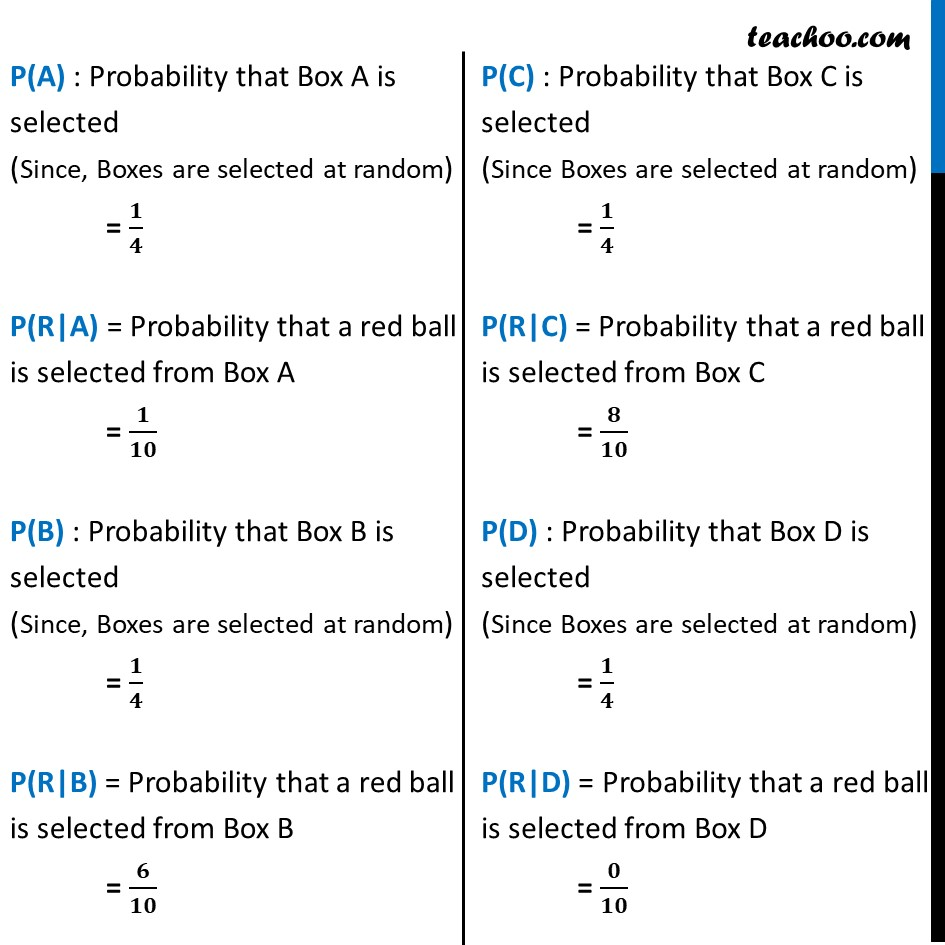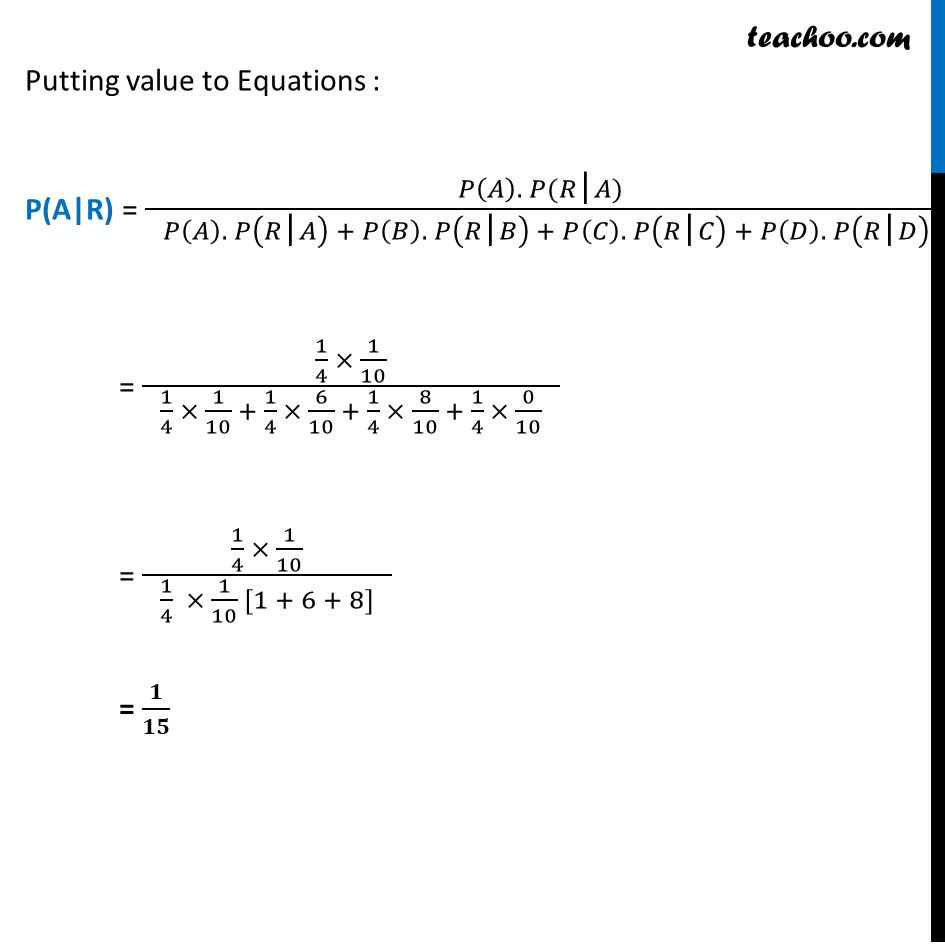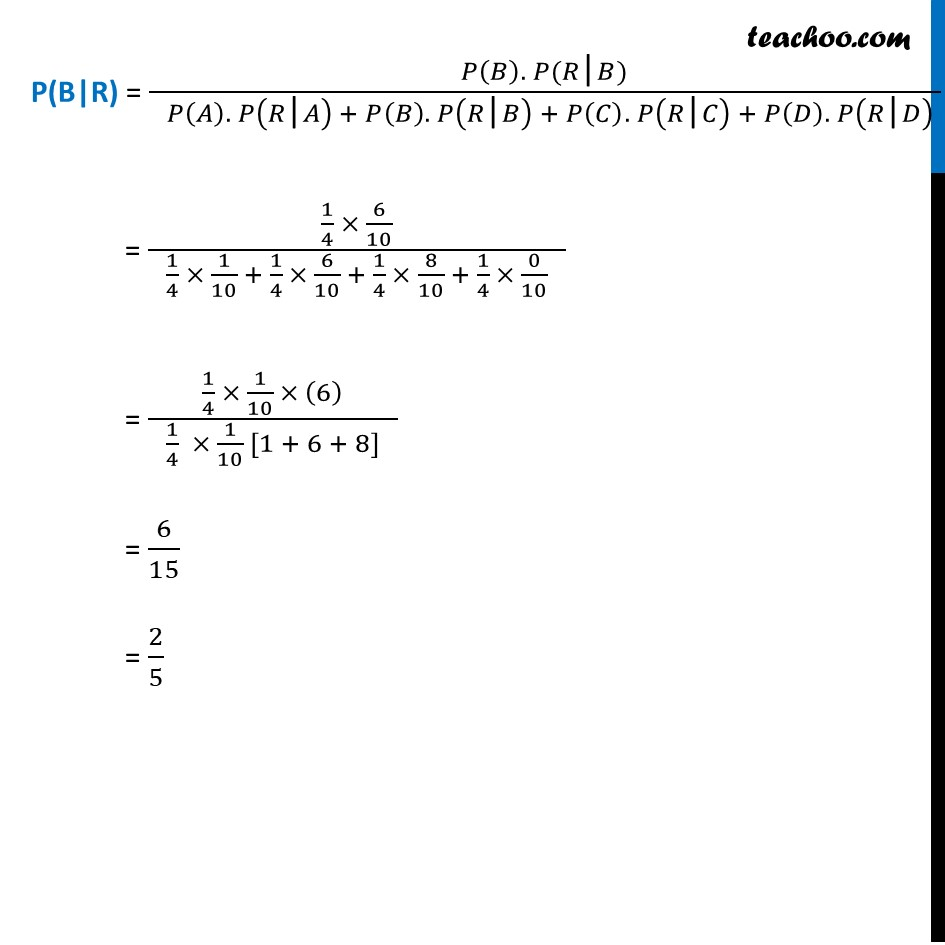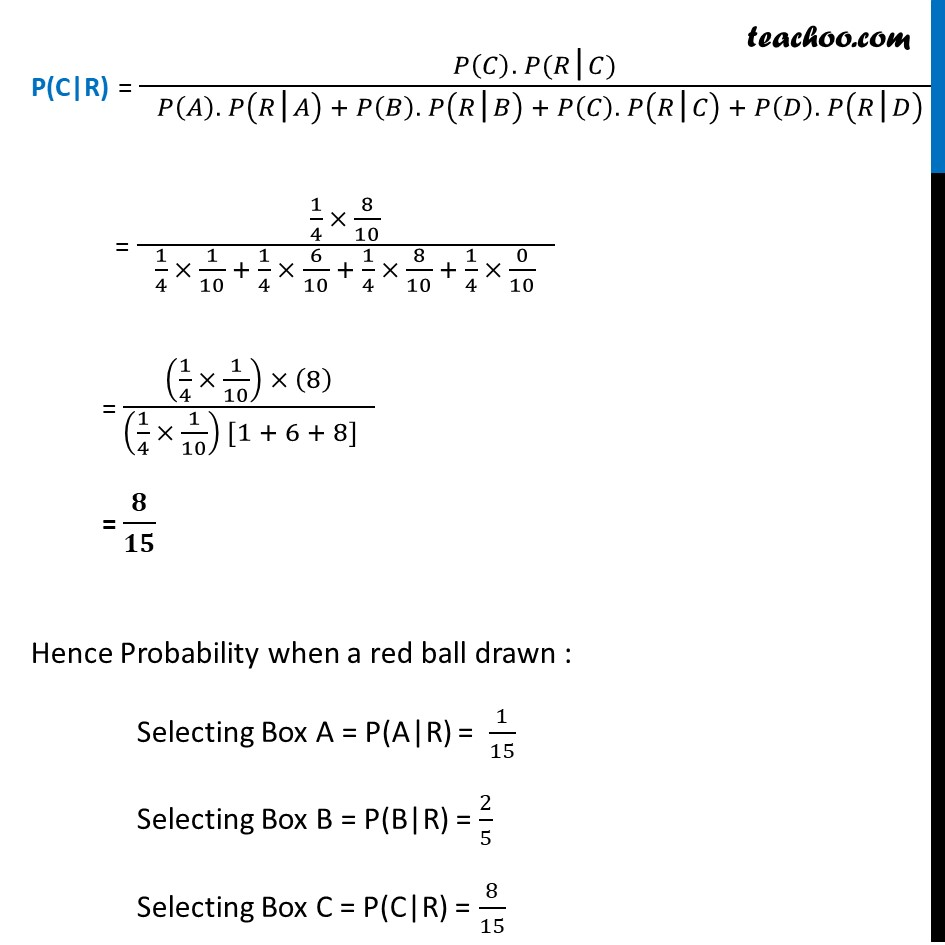Learn in your speed, with individual attention - Teachoo Maths 1-on-1 Class

### Transcript

Misc 6 Suppose we have a four boxes A, B, C and D Containing colored marbles as given below : One of the Boxes has been selected at random and a single marble is drawn from it. If the marble is red, what is the Probability that it was drawn from box A? box B? box C ?Let R : Event that a red marble is drawn A : Event that the marble selected is from Box A B : Event that the marble selected is from Box B C : Event that the marble selected is from Box C D : Event that the marble selected is from Box D We need to find out Probability of drawn a marble is drawn from Box A, if it is a red. i.e. P(A|R) Also We need to find out Probability of drawn a marble is drawn from Box B, if it is a red. i.e. P(B|R) Also We need to find out Probability of drawn a marble is drawn from Box C, if it is a red. i.e. P(C|R) So, P(A|R) = (𝑃(𝐴). 𝑃(𝑅|𝐴))/( 𝑃(𝐴). 𝑃(𝑅|𝐴) + 𝑃(𝐵). 𝑃(𝑅|𝐵) + 𝑃(𝐶). 𝑃(𝑅|𝐶) + 𝑃(𝐷). 𝑃(𝑅|𝐷) ) P(B|R) = (𝑃(𝐵). 𝑃(𝑅|𝐵))/(𝑃(𝐴). 𝑃(𝑅|𝐴) + 𝑃(𝐵). 𝑃(𝑅|𝐵) + 𝑃(𝐶). 𝑃(𝑅|𝐶) + 𝑃(𝐷). 𝑃(𝑅|𝐷) ) P(C|R) = (𝑃(𝐶). 𝑃(𝑅|𝐶))/(𝑃(𝐴). 𝑃(𝑅|𝐴) + 𝑃(𝐵). 𝑃(𝑅|𝐵) + 𝑃(𝐶). 𝑃(𝑅|𝐶) + 𝑃(𝐷). 𝑃(𝑅|𝐷) ) P(A) : Probability that Box A is selected (Since, Boxes are selected at random) = 𝟏/𝟒 P(R|A) = Probability that a red ball is selected from Box A = 𝟏/𝟏𝟎 P(B) : Probability that Box B is selected (Since, Boxes are selected at random) = 𝟏/𝟒 P(R|B) = Probability that a red ball is selected from Box B = 𝟔/𝟏𝟎 P(C) : Probability that Box C is selected (Since Boxes are selected at random) = 𝟏/𝟒 P(R|C) = Probability that a red ball is selected from Box C = 𝟖/𝟏𝟎 P(D) : Probability that Box D is selected (Since Boxes are selected at random) = 𝟏/𝟒 P(R|D) = Probability that a red ball is selected from Box D = 𝟎/𝟏𝟎 Putting value to Equations : P(A|R) = (𝑃(𝐴). 𝑃(𝑅"|" 𝐴))/( 𝑃(𝐴). 𝑃(𝑅"|" 𝐴) + 𝑃(𝐵). 𝑃(𝑅"|" 𝐵) + 𝑃(𝐶). 𝑃(𝑅"|" 𝐶) + 𝑃(𝐷). 𝑃(𝑅"|" 𝐷) ) = (1/4 × 1/10)/( 1/4 × 1/10 + 1/4 × 6/10 + 1/4 × 8/10 + 1/4 × 0/10 ) = (1/4 × 1/10)/( 1/4 × 1/10 [1 + 6 + 8] ) = 𝟏/𝟏𝟓 P(B|R) = (𝑃(𝐵). 𝑃(𝑅"|" 𝐵))/( 𝑃(𝐴). 𝑃(𝑅"|" 𝐴) + 𝑃(𝐵). 𝑃(𝑅"|" 𝐵) + 𝑃(𝐶). 𝑃(𝑅"|" 𝐶) + 𝑃(𝐷). 𝑃(𝑅"|" 𝐷) ) = (1/4 × 6/10)/( 1/4 × 1/10 + 1/4 × 6/10 + 1/4 × 8/10 + 1/4 × 0/10 ) = (1/4 × 1/10 × (6))/( 1/4 × 1/10 [1 + 6 + 8] ) = 6/15 = 2/5 P(C|R) = (𝑃(𝐶). 𝑃(𝑅"|" 𝐶))/( 𝑃(𝐴). 𝑃(𝑅"|" 𝐴) + 𝑃(𝐵). 𝑃(𝑅"|" 𝐵) + 𝑃(𝐶). 𝑃(𝑅"|" 𝐶) + 𝑃(𝐷). 𝑃(𝑅"|" 𝐷) ) = (1/4 × 8/10)/( 1/4 × 1/10 + 1/4 × 6/10 + 1/4 × 8/10 + 1/4 × 0/10 ) = ((1/4 × 1/10) × (8))/((1/4 × 1/10) [1 + 6 + 8] ) = 𝟖/𝟏𝟓 Hence Probability when a red ball drawn : Selecting Box A = P(A|R) = 1/15 Selecting Box B = P(B|R) = 2/5 Selecting Box C = P(C|R) = 8/15